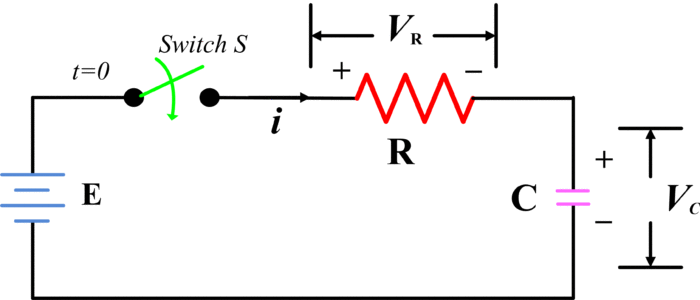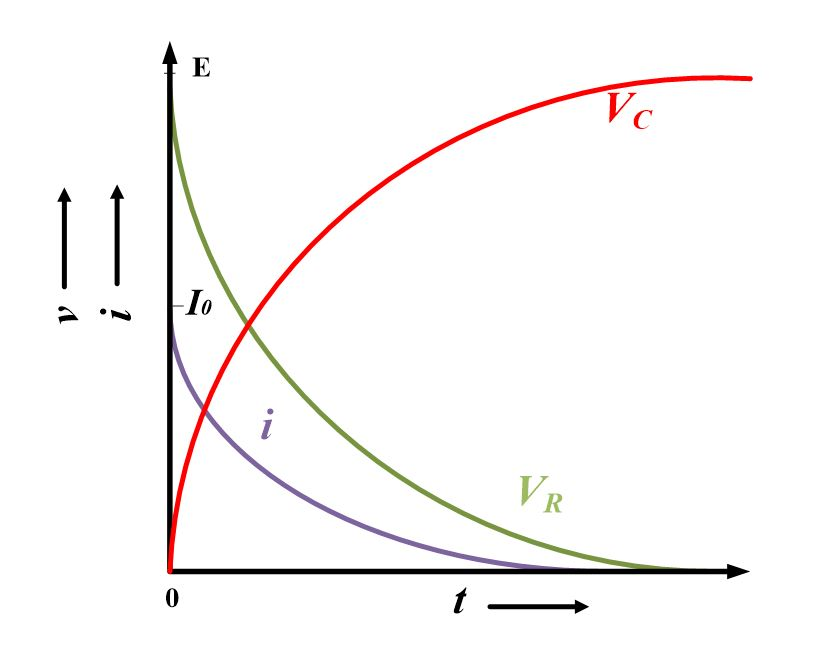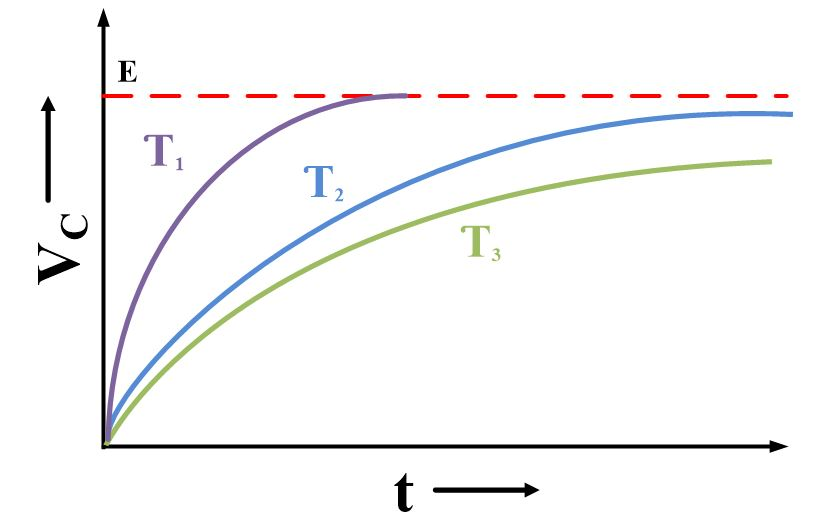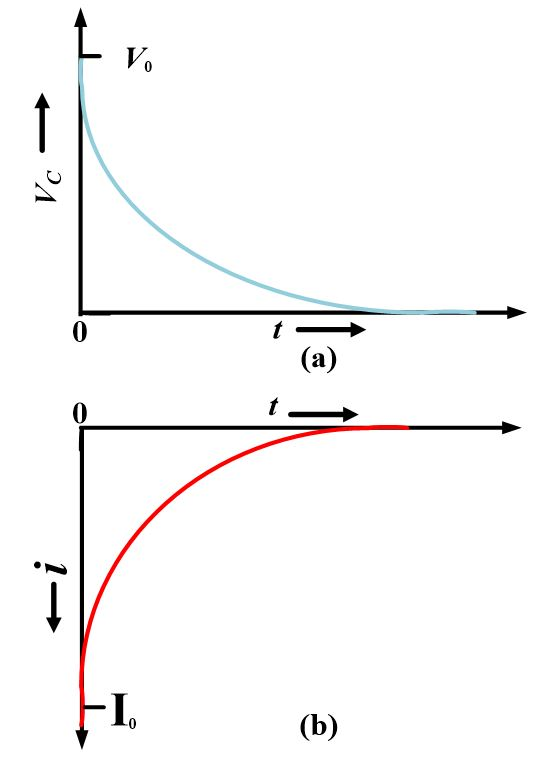Home / Basic Electrical / RC Series Circuit Analysis | RC Time Constant

# RC Series Circuit Analysis | RC Time Constant

Want create site? Find Free WordPress Themes and plugins.

In the case of a resistive-capacitive (RC) series circuit, when the supply is first switched on the charging current is initially at its maximum level, then it gradually falls to zero. The capacitor voltage is zero at first and grows gradually to its maximum level.

As with the RL Circuit, the behavior of an RC circuit can be represented graphically by plotting instantaneous current and voltage versus time. because energy is stored in a charged capacitor, a large current can flow when the capacitor terminals are short-circuited.

An accurate RC series circuit with a source connection is shown in the following Fig.The charging transient begins when a switch is closed, at a time t=0 sec. With the switch closed, the voltage equation is:

$E={{V}_{R}}+{{V}_{c}}$

$E=iR+{{V}_{c}}~~~\cdots ~~\left( 2 \right)$

At the instant, the switch is closed, the capacitor which is considered initially uncharged, has zero voltage (Vc=0), so equation (2) becomes;

$E=iR$

Or

$i={{I}_{o}}=\frac{E}{R}~~~~~~\cdots ~~~~\left( 3 \right)$

The initial charging current Io is then limited by the resistance of the circuit and is determined by the simple application of ohm’s law. On the other hand, after sufficient time, the capacitor is fully charged and no current flows. Then, from eq. (2)

$E=0+{{V}_{c}}~~~~~\cdots \text{ }~~\left( 4 \right)$

Equation (3) and (4) state initial and final conditions for simple charging circuit and it becomes evident to obtain expressions for Vc and i as function of time throughout the transient period. So, we can write eq. (2) as

$E=iR+{{V}_{c}}~~~~~~~~~~~\cdots ~~~~~~~~~~~\left( 5 \right)$

Whereas;

$i=\frac{dq}{dt}$

And

${{V}_{C}}=\frac{q}{C}$

Putting both values in eq. (5) yields

$E=R\frac{dq}{dt}+\frac{q}{C}$

Dividing, both sides by “R”

$\frac{E}{R}=\frac{dq}{dt}+\frac{q}{RC}$

Multiplying both sides by dt

$\frac{E}{R}dt=\frac{dq}{dt}dt+\frac{q}{RC}dt$

$\left( \frac{E}{R}-\frac{q}{RC} \right)dt=dq$

$\frac{dq}{\left( \frac{E}{R}-\frac{q}{RC} \right)}=dt~~~~~~~~~~~~\cdots ~~~~~~~~~~~~\left( 6 \right)$

After integrating eq. (6), we have

$-RC\ln (\frac{E}{R}-\frac{q}{RC})=t+k~~~~~~~~~~~~~~\cdots ~~~~~~~~~~~\left( 7 \right)$

At t=0

$q=0$

$k=-RC\ln \frac{E}{R}$

So,

After putting the the value the of k into eq. (7) we come up with following expression;

$\frac{\ln (\frac{E}{R}-\frac{q}{RC})}{{}^{E}/{}_{R}}=-\frac{t}{RC}$

Taking exponent on both sides yield;

$E-\frac{q}{C}=E{{e}^{-\frac{t}{RC}}}$

$\frac{q}{C}=E-E{{e}^{-\frac{t}{RC}}}$

So final expression for voltage across a capacitor would be;

${{V}_{C}}=E\left( 1-{{e}^{-\frac{t}{RC}}} \right)~~~~~~~~\cdots ~~~~~~~\left( 8 \right)$

Now the voltage across the resistor is;

${{V}_{R}}=E-{{V}_{C}}~~~~~~~~~\cdots ~~~~~~~~~\left( 9 \right)$

Putting value of VC from (8) into (9) yields

${{V}_{R}}=E-E+E{{e}^{-\frac{t}{RC}}}$

${{V}_{R}}=E{{e}^{-\frac{t}{RC}}}$

And

$i=\frac{{{V}_{R}}}{R}=\frac{E}{R}{{e}^{-\frac{t}{RC}}}$

Final expression for current would be,

$i={{I}_{o}}{{e}^{-\frac{t}{RC}}}~~~~~~~~\cdots ~~~~~~~\left( 10 \right)$

The factor of e-t/RC in all the transient equations clearly indicates that current ic and voltage VR approach zero at the same exponential rate as VC approaches E as shown in Fig.The capacitor voltage in a series RC Circuit tends to grow slowly from zero to its final level when the supply voltage is first switched on.

The product RC appearing in the exponential term has the units of seconds.

$RC=\left( ohms \right)\left( farad \right)$

$=\left( \frac{volts}{ampere} \right)\left( \frac{coulombs}{volt} \right)$

$=\left( \frac{volts}{{}^{coulombs}/{}_{second}} \right)\left( \frac{coulombs}{volt} \right)$

$=second$

This product is defined as the time constant of the RC charging circuit and has the symbol 𝝉 (tau)

$\tau =RC$

Since the time constant affects the exponential term, it determines how fast the curve charges. The following figure shows relative effect of  on the transient curve; for larger , the rate of change is less.For t=τ =RC, the exponential factor becomes

${{\varepsilon }^{-1}}=0.368$

So that

${{V}_{C}}=E\left( 1-0.368 \right)=0.632E$

And

$i={{I}_{0}}\left( 0.368 \right)=0.368{{I}_{0}}$

That is; during one time constant, the voltage rises to 63.2 % of its final value and current drops to 36.8 % of its initial value.

# RC Series Circuit Discharging

Discharging is the process of removing charge from a previously charged capacitor with a subsequent delay in capacitor voltage.

When the switch is opened in a series RC Circuit such as that shown in the figure below, there is no effect on the charge stored in the capacitor. The capacitor is able to hold its charge as a static quantity, and its terminal voltage is constant. This contrasts with the case of an inductor, where the charge is a dynamic quantity that cannot be maintained when the current is interrupted.If the switch is initially placed at position 1 the capacitor will charge towards the supply voltage E and after 5-time constants can be considered fully charged.

If the switch is at position 2, the capacitor is across the resistor so that charge leaks through the resistor. The Kirchhoff’s voltage law for discharged case is,

$0=iR+{{V}_{c}}~~~~\cdots ~~~\left( 11 \right)$

Substitute current iC into above equation, we have

$RC\frac{d{{V}_{C}}}{dt}+{{V}_{c}}=0$

So, the final differential equation yields the expression for VC

${{V}_{C}}={{V}_{0}}{{e}^{-\frac{t}{RC}}}$

Similarly, the discharging current can be obtained by solving Eq. (11), which is

$i=-\frac{{{V}_{0}}}{R}{{e}^{-\frac{t}{RC}}}$

The minus sign indicates that current is opposite to that shown in above figure, which is, the discharging current has a direction opposite to that for the charging current. The Vo appearing in the discharging equations is the initial value of the capacitor voltage at the start of the discharge transient. The discharge equations are summarized in the following figure.When a voltage is switched on to a series RC Circuit, Vincreases exponentially toward the level of E over a time of 5RC. The current jumps to I=E/R then decreases exponentially to zero. When the voltage is switched off, Vdecreases exponentially from its maximum level, and I jumps to -E/R then rises exponentially to Zero.

# Energy storage in a capacitor

Capacitance stores energy in an electric field. This energy must come from a source. However, all the energy delivered by the source is not stored in the electric field. The energy stored by the capacitance is only one-half of the total energy delivered by the source. In equitation form, the average energy stored by a capacitance is;

${{W}_{E}}=\frac{1}{2}C{{V}^{2}}~~~~~\cdots ~~~~~\left( 12 \right)$

Where WE represent the average energy stored in an electric field in Joules, C is the value of the capacitance in farads, V is an average value of the voltage across the capacitor in volts.

Eq. (12) is written in terms of average values. This is because it is the average value that is of primary concern, not an instantaneous value. If an AC voltage waveform is impressed across a capacitor, the average energy stored in one cycle is zero. The energy storage feature makes a capacitor useful device for the generation of large current for a very short time.

You May Also Read:

RC Circuit Time Constant using Matlab

Capacitor Charging in RC Circuit using Matlab

Did you find apk for android? You can find new Free Android Games and apps.